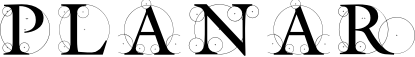#### Previous topic

planar.Vec2Array – 2D Vector Arrays

#### Next topic

planar.Line – Infinite Lines

# planar.Affine – 2D Affine Transforms¶

class planar.Affine

Two dimensional affine transform for linear mapping from 2D coordinates to other 2D coordinates. Parallel lines are preserved by these transforms. Affine transforms can perform any combination of translations, scales/flips, shears, and rotations. Class methods are provided to conveniently compose transforms from these operations.

Internally the transform is stored as a 3x3 transformation matrix. The transform may be constructed directly by specifying the first two rows of matrix values as 6 floats. Since the matrix is an affine transform, the last row is always (0, 0, 1).

Parameters: members (float) – 6 floats for the first two matrix rows.
almost_equals(other)

Compare transforms for approximate equality.

Parameters: other (Affine) – Transform being compared. True if absolute difference between each element of each respective tranform matrix < EPSILON.
classmethod identity()

Return the identity transform.

Return type: Affine
itransform(seq)

Transform a sequence of points or vectors in place.

Parameters: seq – Mutable sequence of Vec2 to be transformed. None, the input sequence is mutated in place.
classmethod rotation(angle, pivot=None)

Create a rotation transform at the specified angle, optionally about the specified pivot point.

Parameters: angle (float) – Rotation angle in degrees pivot (Vec2) – Point to rotate about, if omitted the rotation is about the origin. Affine
classmethod scale(scaling)

Create a scaling transform from a scalar or vector.

Parameters: scaling (float or Vec2) – The scaling factor. A scalar value will scale in both dimensions equally. A vector scaling value scales the dimensions independently. Affine
classmethod shear(x_angle=0, y_angle=0)

Create a shear transform along one or both axes.

Parameters: x_angle (float) – Angle in degrees to shear along the x-axis. y_angle (float) – Angle in degrees to shear along the y-axis. Affine
classmethod translation(offset)

Create a translation transform from an offset vector.

Parameters: offset (Vec2) – Translation offset. Affine
column_vectors

The values of the transform as three 2D column vectors

determinant

The determinant of the transform matrix. This value is equal to the area scaling factor when the transform is applied to a shape.

is_conformal

True if the transform is conformal, i.e., if angles between points are preserved after applying the transform, within rounding limits. This implies that the transform has no effective shear.

is_degenerate

True if this transform is degenerate, which means that it will collapse a shape to an effective area of zero. Degenerate transforms cannot be inverted.

is_identity

True if this transform equals the identity matrix, within rounding limits.

is_orthonormal

True if the transform is orthonormal, which means that the transform represents a rigid motion, which has no effective scaling or shear. Mathematically, this means that the axis vectors of the transform matrix are perpendicular and unit-length. Applying an orthonormal transform to a shape always results in a congruent shape.

is_rectilinear

True if the transform is rectilinear, i.e., whether a shape would remain axis-aligned, within rounding limits, after applying the transform.